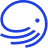# Intro to Impermanent Loss (IL)

## Impermanent Loss can affect your returns in an LP pool.

Impermanent Loss refers to the theoretical loss of value a liquidity provider can experience when pooling their assets.

This phenomenon is dependent on fluctuations in asset prices from the time of deposit to the time of withdrawal, where a higher price fluctuation will be indicative of higher impermanent loss. Impermanent loss is only theoretically “impermanent” because the loss is not realised or made “permanent” until the assets are withdrawn from the pool.

Because an algorithm ensures that the two tokens in any pooled trading pair maintain an equal ratio of 50:50, when these prices fluctuate drastically, a loss in pooled value occurs. The amount of assets that can be withdrawn, as well as the overall dollar value of the position, is lower when in impermanent loss.

This is because a pool’s liquidity is dependent on the ratio between the two asset prices. This ratio is defined by the formula X*Y=K, where assets X and Y result in a reserve amount of liquidity K. Any change in K results in impermanent loss felt by liquidity providers. For instance, if the ratio of pooled assets decreases from 10:1 to 5:1 because one asset increases in price (or the other asset decreases in price), the divergence will necessitate a rearranging of funds to maintain a balanced pool.

Let’s look at a specific example of the above change: a liquidity provider enters \$100k into the THORChain BTC: RUNE pool when the BTC price is \$50,000 and RUNE price is \$10. They, therefore, entered with 1 BTC and 5,000 RUNE; thus, a ratio of 5000:1. If the BTC price remains the same while the RUNE price increases to \$50, one would assume the value of the pair would be \$300k (\$50k +\$250k).

However, the new price ratio is now 1,000:1, and the price ratio change is 5x or 500%. Because the pair must retain a 50:50 value balance, the algorithm will rearrange the pooler’s asset amounts to 2.235 BTC and 2235 Rune, making the actual LP share value \$223,500. The depositor, therefore, incurs an impermanent loss amounting to \$76,500, or 25.5%. They still have a claim to their share but at 25.5% less dollar value than they would have by simply holding. Again, this loss is not made “permanent” until the assets are withdrawn from the pool.

So, why would someone bother with LP if you always incur IL? The answer is threefold:

1. You are earning trade fees and block rewards while experiencing IL and hope that earnings will more than compensate for the IL.
2. With THORChain specifically, there is IL Protection (ILP) for LPs based on the length of the deposit.
3. Timing price movements in liquidity pools can be used to one’s advantage to obtain more of the asset that isn’t gaining dollar value by selling the paired asset “on the way up”.### Need Support?

Can't find what you're looking for? File a "SOS Help-Desk" ticket in our Discord
Contact Support